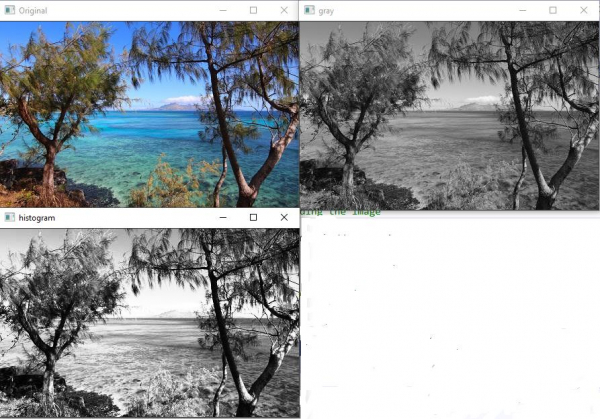# How to apply Histogram Equalizer in OpenCV using C++?

The histogram represents the depth intensity of an image. For example, consider an image with a color depth of 8 bit. It means every pixel can have color depth from 0 to  Means from 0 to 255. If the image is an RGB image, it has a red, green, and blue channel. For example, at the point of the image, there is only red. Then the color depth of that image is in the red channel, and the value of the pixel will vary from 0 to 255. 0 means no red and 255 means more read.

The histogram shows this kind of result for all of the channels and all of the colors. By changing the value of the pixels, you can change the intensity of some specific color pixels. For example, if you want to increase the red channel density, you can change the red channel's value.

First of all, we will apply histogram equalizer in a gray image. A gray image includes only black and white. That means there is only one channel. The image is an 8-bit image. It means the color depth varies from 0 to 255. 0 means white, and 255 means black. In the following code, we will load a color image and will convert it to grayscale. Then we will use the 'equalizeHist' function of OpenCV to equalize the pixel values.

The following program shows how to apply Histogram Equalizer in OpenCV.

## Example

#include<opencv2/highgui/highgui.hpp>
#include<opencv2/imgproc/imgproc.hpp>
using namespace cv;
using namespace std;
int main() {
Mat original;//Declaring a matrix//
Mat gray;//declaring a matrix//
cvtColor(original, gray, COLOR_BGR2GRAY);//converting to grayscale//
Mat hist;//declaring a matrix//
equalizeHist(gray, hist);//applying histogram equalizer
namedWindow("Original");//window for actual image//
namedWindow("gray");//window for grayscale image//
namedWindow("histogram");//window for histogram//
imshow("Original", original);//showing actual image//
imshow("gray", gray);//showing grayscale image//
imshow("histogram", hist);//showing histogram effect
waitKey(0);//wait for keystroke//
return(0);
}

## Output